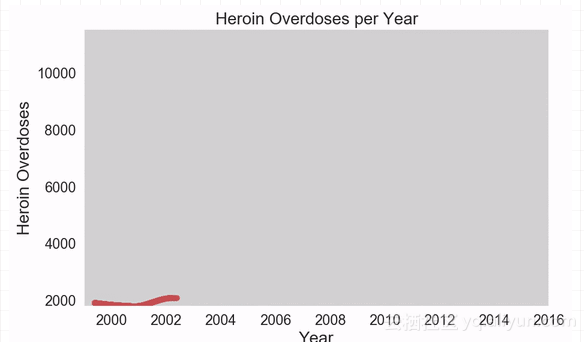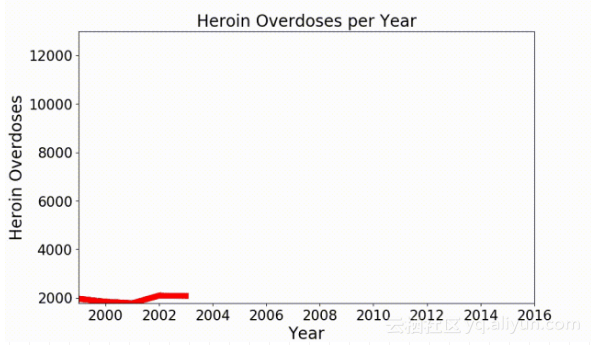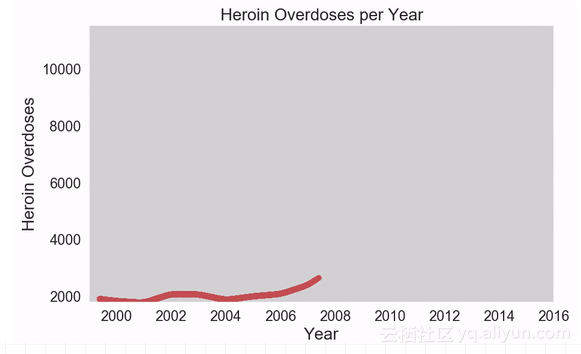# matplotlib秘技：让可视化图形动起来技术小能手 2018-11-23Python的matplotlib和seaborn是非常好用的绘图库。但它们创建的都是静态图像，难以通过动态、美观的方式描述数据值的变化。如果你的下一次演示或者下一篇博客文章，能用动态图形展示数据的发展，该有多好？更妙的是，你可以继续使用matplotlib、seaborn或者其他你喜欢用的库。

1. `import numpy as np`

2. `import pandas as pd`

3. `import seaborn as sns`

4. `import matplotlib`

5. `import matplotlib.pyplot as plt`

6. `import matplotlib.animation as animation`

1. `overdoses = pd.read_excel('overdose_data_1999-2015.xls',sheetname='Online',skiprows =6)`

2. `def get_data(table,rownum,title):`

3. ` data = pd.DataFrame(table.loc[rownum][2:]).astype(float)`

4. ` data.columns = {title}`

5. ` return data`

1. `%matplotlib notebook`

2. `title = 'Heroin Overdoses'`

3. `d = get_data(overdoses,18,title)`

4. `x = np.array(d.index)`

5. `y = np.array(d['Heroin Overdoses'])`

6. `overdose = pd.DataFrame(y,x)`

7. `#XN,YN = augment(x,y,10)`

8. `#augmented = pd.DataFrame(YN,XN)`

9. `overdose.columns = {title}`

1. `Writer = animation.writers['ffmpeg']`

2. `writer = Writer(fps=20, metadata=dict(artist='Me'), bitrate=1800)`

1. `fig = plt.figure(figsize=(10,6))`

2. `plt.xlim(1999, 2016)`

3. `plt.ylim(np.min(overdose), np.max(overdose))`

4. `plt.xlabel('Year',fontsize=20)`

5. `plt.ylabel(title,fontsize=20)`

6. `plt.title('Heroin Overdoses per Year',fontsize=20)`

1. `def animate(i):`

2. ` data = overdose.iloc[:int(i+1)] # 选定数据范围`

3. ` p = sns.lineplot(x=data.index, y=data[title], data=data, color="r")`

4. ` p.tick_params(labelsize=17)`

5. ` plt.setp(p.lines,linewidth=7)`

1. `ani = matplotlib.animation.FuncAnimation(fig, animate, frames=17, repeat=True)`

1. `ani.save('HeroinOverdosesJumpy.mp4', writer=writer)`1. `def augment(xold,yold,numsteps):`

2. ` xnew = []`

3. ` ynew = []`

4. ` for i in range(len(xold)-1):`

5. ` difX = xold[i+1]-xold[i]`

6. ` stepsX = difX/numsteps`

7. ` difY = yold[i+1]-yold[i]`

8. ` stepsY = difY/numsteps`

9. ` for s in range(numsteps):`

10. ` xnew = np.append(xnew,xold[i]+s*stepsX)`

11. ` ynew = np.append(ynew,yold[i]+s*stepsY)`

12. ` return xnew,ynew`

1. `def smoothListGaussian(listin,strippedXs=False,degree=5): `

2. ` window=degree*2-1 `

3. ` weight=np.array([1.0]*window) `

4. ` weightGauss=[] `

5. ` for i in range(window): `

6. ` i=i-degree+1 `

7. ` frac=i/float(window) `

8. ` gauss=1/(np.exp((4*(frac))**2)) `

9. ` weightGauss.append(gauss)`

10. ` weight=np.array(weightGauss)*weight `

11. ` smoothed=[0.0]*(len(listin)-window) `

12. ` for i in range(len(smoothed)): smoothed[i]=sum(np.array(listin[i:i+window])*weight)/sum(weight) `

13. ` return smoothe`

1. `sns.set(rc={'axes.facecolor':'lightgrey', 'figure.facecolor':'lightgrey','figure.edgecolor':'black','axes.grid':False})`AI派”。

5698人浏览
2020-07-13

1249人浏览
2017-08-01 16:19:00
Matplotlib入门
1430人浏览
2018-07-30 10:54:00

5586人浏览
2016-12-23 17:25:26

1416人浏览
2017-05-31 11:00:00

1154人浏览
2017-08-01 14:47:00
Matplotlib 中文用户指南 1 简介
766人浏览
2017-01-30 11:38:40

1872人浏览
2017-04-10 06:49:38
0
1
2715